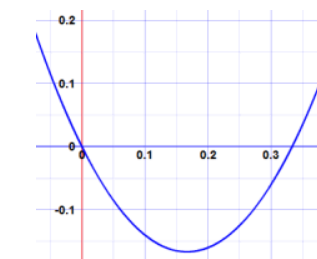# What does it meaning to plot?

In mathematics, to plot means to draw a graph of some data that is given.

A plot is a graphical technique for representing a data set, usually as a graph showing the relationship between two or more variables.

To plot means to draw on a graph or map.

For example, we can plot the point (10,5) on a graph paper

We can also plot a quadratic function to get a parabola on a graph as shown below.Updated on: 10-Oct-2022

13 Views